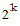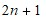# NMath User's Guide

13.2 Numerical Integration (.NET, C#, CSharp, VB, Visual Basic, F#)

Numerical integration, also called quadrature, computes an approximation of the integral of a function over some interval. There are many methods for numerically evaluating integrals. NMath provides two of the most widely used, general purpose families of methods: Romberg integration, and Gauss-Kronrod integration.

NOTE—Class Polynomial provides a method for constructing the exact antiderivative of a polynomial. See Section 13.4 for more information.

Computing Integrals

The Integrate() method on OneVariableFunction (Section 13.1) computes the integral of a function over a given interval. For example, if f is a OneVariableFunction, this code integrates f over the interval -1 to 1:

Code Example – C# calculus

```double integral = f.Integrate( -1, 1 );
```

Code Example – VB calculus

```Dim Integral As Double = F.Integrate(-1, 1)
```

NOTE—NMath does not directly support improper intervals; that is, it must be possi­ble to evaluate the function at both the lower and upper bounds, and at any point in between (no singularities).

To perform integration, every OneVariableFunction has an object associated with it. NMath integration classes such as and implement the IIntegrator interface. The default integrator for a OneVariableFunction is an instance of RombergIntegrator, which may be changed using the Integrator property. Thus:

Code Example – C# calculus

```f.Integrator = new GaussKronrodIntegrator();
```
```double integral = f.Integrate( 0, Math.PI );
```

Code Example – VB calculus

```F.Integrator = New GaussKronrodIntegrator()
```
```Dim Integral As Double = F.Integrate(0, Math.PI)
```

You can also change the default IIntegrator associated with all instances of OneVariableFunction using the static DefaultIntegrator property. For instance:

Code Example – C# calculus

```OneVariableFunction.DefaultIntegrator =
```
```  new GaussKronrodIntegrator();

```

```var d = new Func<double, double>( MyFunction );
```
```var f = new OneVariableFunction( d );

```

```double integral = f.Integrate( 0, 1 );  // uses Gauss-Kronrod

```

Code Example – VB calculus

```OneVariableFunction.DefaultIntegrator = New
GaussKronrodIntegrator()

```

```Dim D As New Func(Of Double, Double)(AddressOf MyFunction)
```
```Dim F As New OneVariableFunction(D)

```

```Dim Integral As Double = f.Integrate(0, 1) ' uses Gauss-Kronrod
```

Romberg Integration

In general, the class of methods known as Newton-Cotes formulas estimate the integral of a function over a given interval by dividing the interval intopanels, where k is called the order, estimating the integral within each panel, then summing the estimates. For instance, the trapezoidal rule approximates the function in each panel by a straight line between the end points. Simpson's rule approximates the function in two adjacent panels by a quadratic function connecting the two outer points and the common midpoint. Higher-level methods are obtained by interpolating higher degree polynomial segments.

Because all methods evaluate the function at the same set of points, higher-level approximations can be derived from lower-level approximations. For example, it can be shown that a kth-order Simpson's rule approximation can be derived from two trapezoidal rule approximations of order k and k-1. Similarly, a Boole's rule approximation, which fits third-degree polynomials through the points associated with four-panel partitions of the interval, can be derived from two Simpson's rule approximations of order k and k-1. In this way, all higher level approximations can be derived from a series of trapezoidal rule approximations.

This iterated application of trapezoidal rule approximations is known as Romberg integration. Romberg integration is a very powerful method for quickly and accurately integrating smooth functions.

In NMath, instances of class compute successive Romberg approximations of increasing order until the estimated error in the approximation is less than a specified error tolerance, or until the maximum order is reached. The default error tolerance is 1e-8, and the default maximum order is 20.

To perform integration, every OneVariableFunction has an object associated with it, which is used by the Integrate() method to compute integrals. The default for a OneVariableFunction is an instance of RombergIntegrator. For example, assuming f is a OneVariableFunction, this code uses the default RombergIntegrator to integrate over the interval -1 to 1:

Code Example – C# calculus

```double estimate = f.Integrate( -1, 1);
```

Code Example – VB calculus

```Dim Estimate As Double = f.Integrate(-1, 1)
```

The underlying IIntegrator can be accessed using the Integrator property.

In some cases, you may wish to create a RombergIntegrator yourself. This gives you more control over the integration process, and allows you to reuse a customized integrator to integrate several functions, or one function over several intervals. Thus, this code instantiates a RombergIntegrator, uses the provided Tolerance property to change the error tolerance and the MaximumOrder property to change the maximum order, then calls the Integrate() method on RombergIntegrator to integrate functions f and g:

Code Example – C# calculus

```var rom = new RombergIntegrator();
```
```rom.Tolerance = 1e-6;
```
```rom.MaximumOrder = 16;
```
```double integralF = rom.Integrate( f, -1, 1);
```
```double integralG = rom.Integrate( g, 0, 2 * Math.PI );
```

Code Example – VB calculus

```Dim Rom As New RombergIntegrator()
```
```Rom.Tolerance = "1e-6"
```
```Rom.MaximumOrder = 16
```
```Dim IntegralF As Double = Rom.Integrate(f, -1, 1)
```
```Dim IntegralG As Double = Rom.Integrate(g, 0, 2 * Math.PI)
```

To compute a Romberg estimate of a specific order, k, you can also set the MaximumOrder to k and the Tolerance to a negative value. This code configures the RombergIntegrator to compute an 8th-order approximation:

Code Example – C# calculus

```var rom = new RombergIntegrator();
```
```rom.Tolerance = -1;
```
```rom.MaximumOrder = 8;
```
```double estimate = rom.Integrate( f, -1, 1);
```

Code Example – VB calculus

```Dim Rom = New RombergIntegrator()
```
```Rom.Tolerance = -1
```
```Rom.MaximumOrder = 8
```
```Dim Estimate As Double = Rom.Integrate(f, -1, 1)
```

After computing an estimate, a RombergIntegrator holds a record of the iteration process. Read-only properties are provided for accessing this information:

RombergEstimate gets the Romberg estimate for the integral, as returned by the Integrate() method.

RombergErrorEstimate gets an estimate of the error in the Romberg estimate of the integral just computed.

ToleranceMet returns true if the estimate of the error in the Romberg approximation just computed is less than or equal to the tolerance; otherwise, false. (Integration ends either when the estimated error in the approximation is less than tolerance, or when the maximum order is reached.)

Order gets the order of the Romberg approximation just computed.

TrapeziodEstimate gets the estimate for the integral yielded by the compound trapeziod rule where the number of panels is equal to the order of the Romberg estimate.

SimpsonEstimate gets the estimate for the integral yielded by the compound Simpson's rule where the number of panels is equal to the order of the Romberg estimate. (Note: Returns 0 if Order = 0.)

Tableau gets the entire DoubleMatrix of successive approximations computed while computing a Romberg estimate. The rows are the order of approximation. The columns are the level of approximation. The first column contains the trapezoidal approximations, the second column the Simpson's rule approximations, the third column the Boole's rule approximations, and so on, up to the Order of the approximation just computed.

Thus, this code retrieves the Boole's rule approximation:

Code Example – C# calculus

```var rom = new RombergIntegrator();
```
```double integral = rom.Integrate( f, 0, 1);
```
```int order = rom.Order;
```
```DoubleMatrix tableau = rom.Tableau;

```

```double boole;
```
```if ( order >= 2 )
```
```{
```
```  boole = tableau[ order, 2 ];
```
```}
```

Code Example – VB calculus

```Dim Rom As New RombergIntegrator()
```
```Dim Integral As Double = Rom.Integrate(f, 0, 1)
```
```Dim Order As Integer = Rom.Order
```
```Dim Tableau As DoubleMatrix = Rom.Tableau

```

```Dim Boole As Double
```
```If Order >= 2 Then
```
```  Boole = Tableau(Order, 2)
```
```End If
```

Gauss-Kronrod Integration

Gaussian integration estimates an integral by evaluating the function at non-equally spaced points over the interval. The method attempts to pick optimal points at which to evaluate the function, and furthermore to weight the contribution of each point. Gauss-Kronrod integration is an adaptive Gaussian quadrature method in which the function is evaluated at special points known as Kronrod points. The Gauss-Kronrod method is especially suited for non-singular oscillating integrands.

NMath includes Gauss-Kronrod classes for different numbers of Kronrod points (, beginning with a Gauss 10-point rule):

GaussKronrod21Integrator approximates integrals using the Gauss 10-point and the Kronrod 21-point rule.

GaussKronrod43Integrator approximates integrals using the Gauss 21-point and the Kronrod 43-point rule.

GaussKronrod87Integrator approximates integrals using the Gauss 43-point and the Kronrod 87-point rule.

Finally, the automatic class uses Gauss-Kronrod rules with increasing number of points. Approximation ends when the relative error is less than the tolerance scaled by the integration result, or when the maximum number of points is reached. The default error tolerance is 1e-7; the default maximum number of points is 87. Unless you have reason to believe in advance that a particular Gauss-Kronrod rule is optimal for your function, it is recommended that you use the automatic integrator.

By default, OneVariableFunction objects use RombergIntegrator objects to compute integrals, but this may be changed using the Integrator property. For instance:

Code Example – C# calculus

```f.Integrator = new GaussKronrodIntegrator();
```
```double integral = f.Integrate( 0, Math.PI );
```

Code Example – VB calculus

```F.Integrator = New GaussKronrodIntegrator()
```
```Dim Integral As Double = F.Integrate(0, Math.PI)
```

This code specifically uses the GaussKronrod43Integrator, rather than the automatic GaussKronrodIntegrator:

Code Example – C# calculus

```f.Integrator = new GaussKronrod43Integrator();
```
```double integral = f.Integrate( -1, 1 );
```

Code Example – VB calculus

```F.Integrator = New GaussKronrod43Integrator()
```
```Dim Integral As Double = F.Integrate(-1, 1)
```

In some cases you may wish to create a Gauss-Kronrod integrator yourself. This gives you more control over the integration process, and allows you to reuse a customized integrator to integrate several functions, or one function over several intervals. Thus, this code instantiates a , uses the provided Tolerance property to change the error tolerance, then calls the Integrate() method on to integrate functions f and g:

Code Example – C# calculus

```var gk = new GaussKronrodIntegrator();
```
```gk.Tolerance = 1e-6;
```
```double integralF = gk.Integrate( f, -1, 1);
```
```double integralG = gk.Integrate( g, 0, 2 * Math.PI );
```

Code Example – VB calculus

```Dim GK As New GaussKronrodIntegrator()
```
```GK.Tolerance = "1e-6"
```
```Dim IntegralF As Double = GK.Integrate(f, -1, 1)
```
```Dim IntegralG As Double = GK.Integrate(g, 0, 2 * Math.PI)
```

Read-only properties are provided for accessing information about an integral approximation, once it has been computed:

RelativeErrorEstimate gets an estimate of the relative error for the integral approximation.

ToleranceMet gets a boolean value indicating whether or not the relative error for the integral approximation is less than the tolerance scaled by the integration result.

PreviousEstimate gets the integral approximation calculated using the previous rule—for example, the Gauss 10-point rule for a GaussKronrod21Integrator, the Kronrod 21-point rule for a GaussKronrod43Integrator, and so forth.

For instance, this code checks whether the error tolerance was met before proceeding:

Code Example – C# calculus

```var gk = new GaussKronrodIntegrator();
```
```gk.Tolerance = 1e-6;
```
```double integral = gk.Integrate( f, -1, 1 );

```

```if ( gk.ToleranceMet )
```
```{
```
```  // Do something here...
```
```}
```

Code Example – VB calculus

```Dim GK As New GaussKronrodIntegrator()
```
```GK.Tolerance = "1e-6"
```
```Dim Integral As Double = GK.Integrate(f, -1, 1)

```

```If GK.ToleranceMet Then
```
```  ' Do something here...
```
```End If
```

Top

Top